Скачать презентацию Specific Heat Capacity Thermal Physics Lesson 1

201e67bed8822de248996bf193f77315.ppt

• Количество слайдов: 14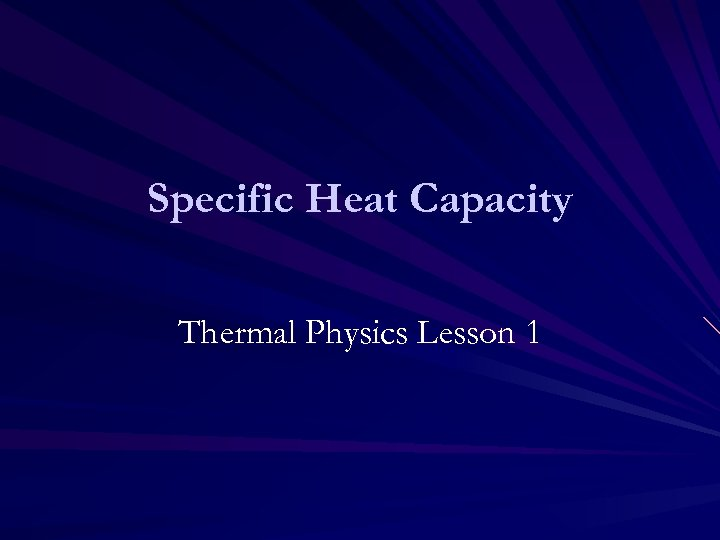Specific Heat Capacity Thermal Physics Lesson 1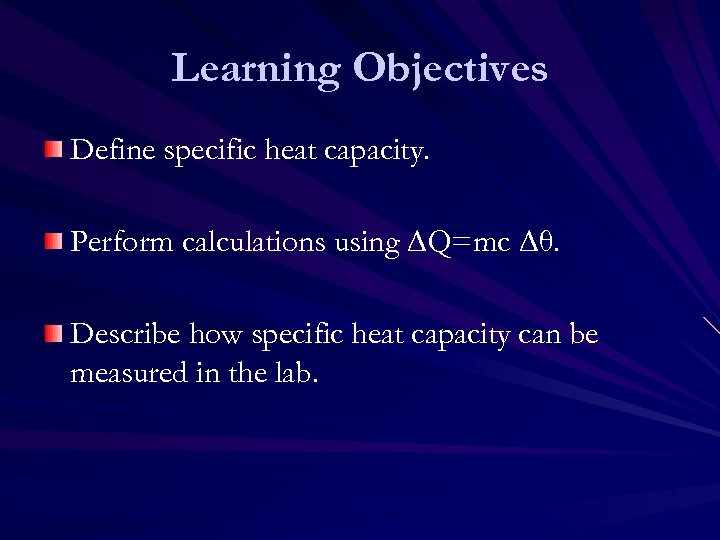Learning Objectives Define specific heat capacity. Perform calculations using ∆Q=mc ∆θ. Describe how specific heat capacity can be measured in the lab.Homework Complete worksheet to practice calculations we will look at today.Why do we care The large SHC of water is particularly important for the development and maintenance of life on Earth.Why are we learning this? Historical Context Images of Joule, Kelvin Steam engines Thermal physics in stars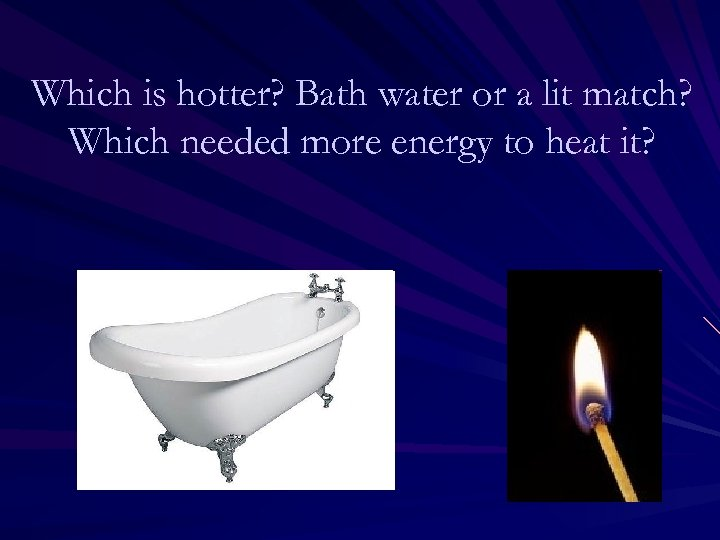Which is hotter? Bath water or a lit match? Which needed more energy to heat it?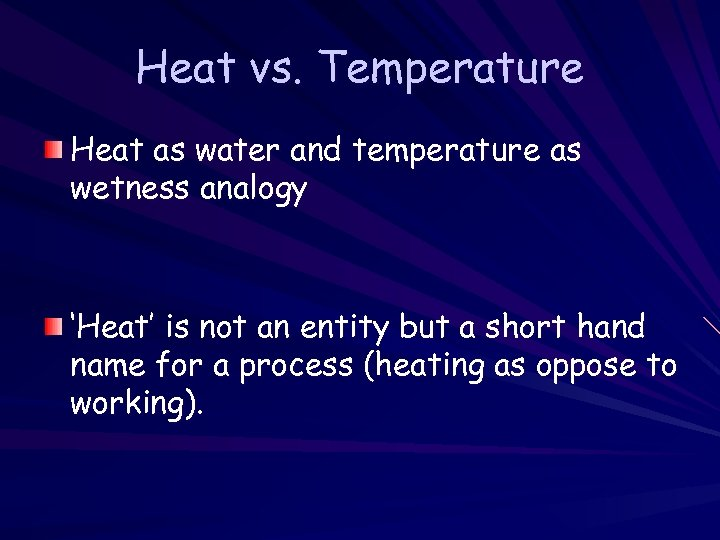Heat vs. Temperature Heat as water and temperature as wetness analogy ‘Heat’ is not an entity but a short hand name for a process (heating as oppose to working).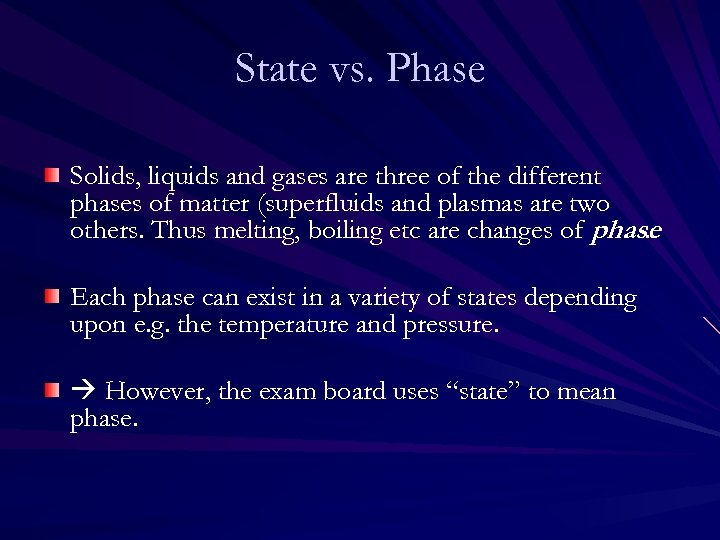State vs. Phase Solids, liquids and gases are three of the different phases of matter (superfluids and plasmas are two others. Thus melting, boiling etc are changes of phase. Each phase can exist in a variety of states depending upon e. g. the temperature and pressure. However, the exam board uses “state” to mean phase.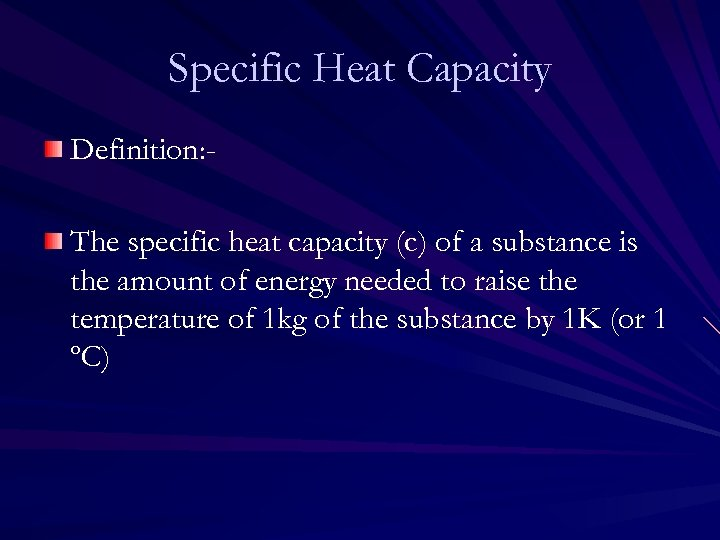Specific Heat Capacity Definition: The specific heat capacity (c) of a substance is the amount of energy needed to raise the temperature of 1 kg of the substance by 1 K (or 1 ºC)Specific Heat Capacity Equation: where: ∆Q is the energy change in J m is the mass in kg c is the specific heat capacity in J K-1 kg-1 ∆θ is the temperature change in K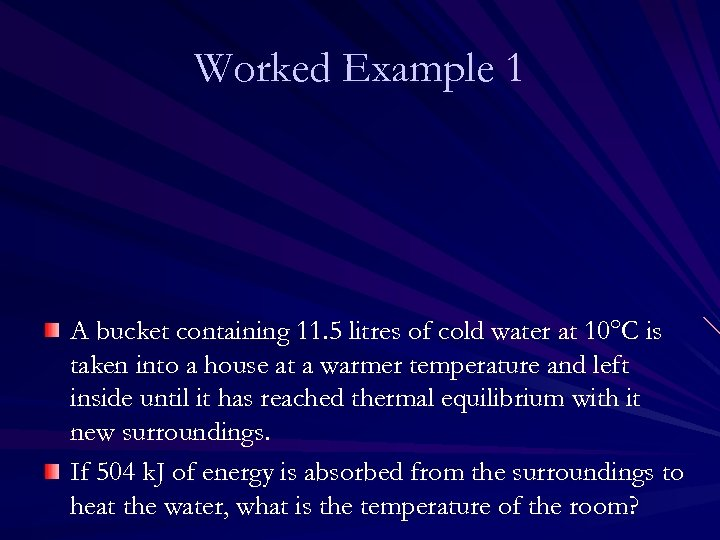Worked Example 1 A bucket containing 11. 5 litres of cold water at 10°C is taken into a house at a warmer temperature and left inside until it has reached thermal equilibrium with it new surroundings. If 504 k. J of energy is absorbed from the surroundings to heat the water, what is the temperature of the room?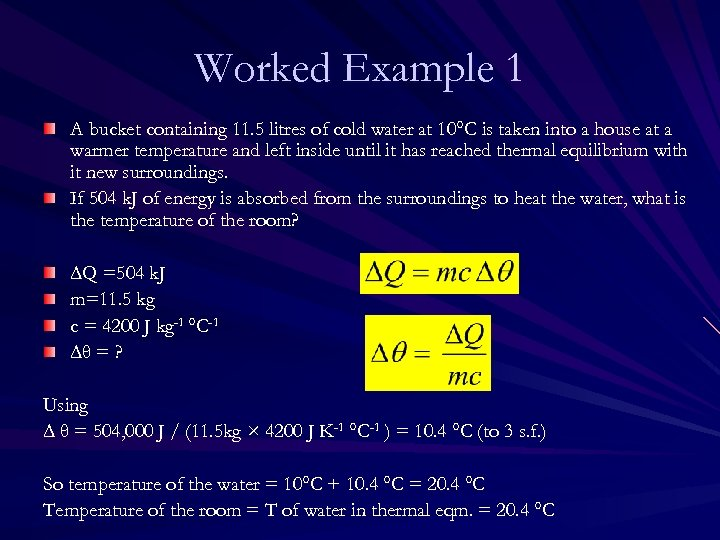Worked Example 1 A bucket containing 11. 5 litres of cold water at 10°C is taken into a house at a warmer temperature and left inside until it has reached thermal equilibrium with it new surroundings. If 504 k. J of energy is absorbed from the surroundings to heat the water, what is the temperature of the room? ∆Q =504 k. J m=11. 5 kg c = 4200 J kg-1 °C-1 ∆θ = ? Using ∆ θ = 504, 000 J / (11. 5 kg × 4200 J K-1 °C-1 ) = 10. 4 °C (to 3 s. f. ) So temperature of the water = 10°C + 10. 4 °C = 20. 4 °C Temperature of the room = T of water in thermal eqm. = 20. 4 °C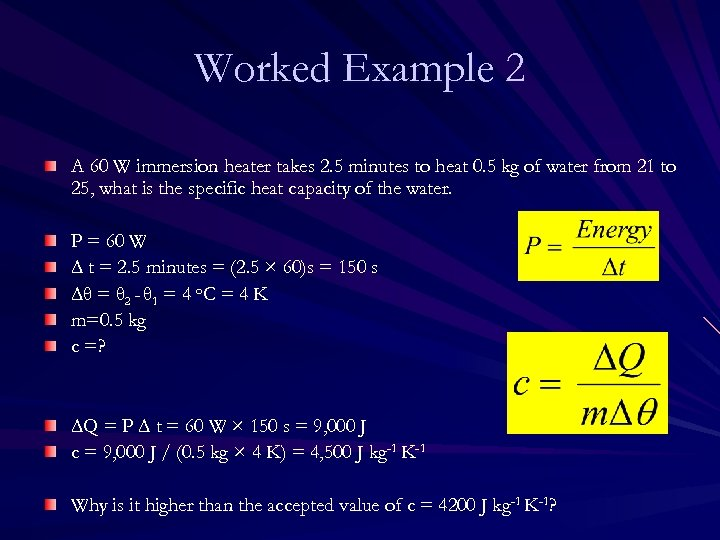Worked Example 2 A 60 W immersion heater takes 2. 5 minutes to heat 0. 5 kg of water from 21 to 25, what is the specific heat capacity of the water. P = 60 W ∆ t = 2. 5 minutes = (2. 5 × 60)s = 150 s ∆θ = θ 2 - θ 1 = 4 o. C = 4 K m=0. 5 kg c =? ∆Q = P ∆ t = 60 W × 150 s = 9, 000 J c = 9, 000 J / (0. 5 kg × 4 K) = 4, 500 J kg-1 K-1 Why is it higher than the accepted value of c = 4200 J kg-1 K-1?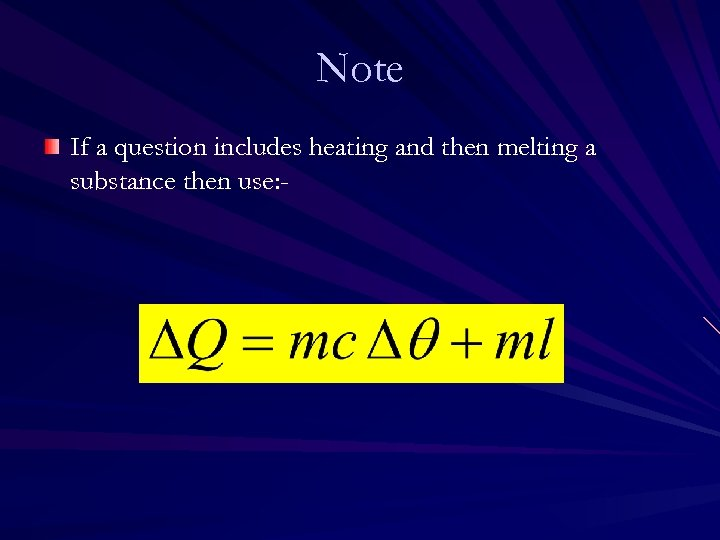Note If a question includes heating and then melting a substance then use: -